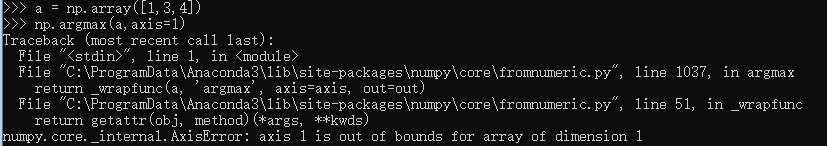• ## LaTeX argmin argmax 下标使用方法

万次阅读 多人点赞 2015-11-25 13:51:16
LaTeX中，使用argmin，argmax下标。

本系列文章由 @yhl_leo 出品，转载请注明出处。
文章链接：  http://blog.csdn.net/yhl_leo/article/details/50036001

LaTeX中，使用argmin，argmax时，设置下标可用下面方法：
\begin{equation}
\mathop{\arg\min}_{\theta} \ \ \| \mathrm{J} (\theta)\|.
\end{equation}

arg⁡min⁡θ  ∥J(θ)∥.
\mathop{\arg\min_{\theta}} \ \ \| \mathrm{J} (\theta)\|.


展开全文latex
• ## argmin ，argmax函数

万次阅读 2018-05-12 10:16:18
在数学中，ARG MAX（或ARGMAX）代表最大值，即给定参数的点集，给定表达式的值达到其最大值：换一种说法，是f（x）具有最大值M的x的值的集合。例如，如果f（x）是1- | x |，那么它在x = 0时达到其最大值1并且仅在...
在数学中，ARG MAX（或ARGMAX）代表最大值，即给定参数的点集，给定表达式的值达到其最大值：$\underset{x}{\operatorname{arg\,max}} \, f(x) := \{x\ |\ \forall y : f(y) \le f(x)\}$换一种说法，$\underset{x}{\operatorname{arg\,max}} \, f(x)$是f（x）具有最大值M的x的值的集合。例如，如果f（x）是1- | x |，那么它在x = 0时达到其最大值1并且仅在那里， 所以$\underset{x}{\operatorname{arg\,max}} \, (1-|x|) = \{0\}$.$\underset{x}{\operatorname{arg\,max}} \, (1-|x|) = \{0\}$.等价地，如果M是f的最大值，那么arg max是最大值的水平集$\underset{x}{\operatorname{arg\,max}} \, f(x) = f^{-1}(M) = \{x\ |\ f(x) = M \}$如果最大值达到一个值，那么一个将该点称为arg max，这意味着我们将arg max定义为一个点，而不是一组点。所以，例如$\underset{x\in \Bbb{R}}{\operatorname{arg\,max}} (x(10-x)) = 5$（而不是单独集合{5}），因为x（10-x）的最大值是25，这在x = 5时发生。 但是，如果在许多值处达到最大值，则arg max是一组点。 然后，我们举例说$\underset{x \in [0,4\pi]}{\operatorname{arg\,max}} \, \cos(x) = \{0,2\pi,4\pi\}$因为cos（x）的最大值是1，当x =0,2π或4π时，这个间隔发生。在整个实线上，arg max是$\{0, 2\pi, -2\pi, 4\pi, \dots \}.$arg min（或argmin）被类似地定义.还要注意函数通常不会达到最大值，因此通常不会有arg max:$\underset{x\in \Bbb{R}}{\operatorname{arg\,max}}\, x$ 没有定义，因为x在实线上是无界的。然而，通过极值定理（或经典致密性理论），紧凑区间上的连续函数具有最大值，因此arg max。argmin 表示使目标函数取最小值时的变量值翻译于：http://www.cppblog.com/guijie/archive/2010/12/13/136273.html
展开全文• ## tf.argmax()解析

万次阅读 多人点赞 2018-07-27 17:00:53
tf.argmax(input,axis)根据axis取值的不同返回每行或者每列最大值的索引。 这个很好理解，只是tf.argmax()的参数让人有些迷惑，比如，tf.argmax(array, 1)和tf.argmax(array, 0)有啥区别呢？ 这里面就涉及到一个概念...
tf.argmax(input,axis)根据axis取值的不同返回每行或者每列最大值的索引。 这个很好理解，只是tf.argmax()的参数让人有些迷惑，比如，tf.argmax(array, 1)和tf.argmax(array, 0)有啥区别呢？ 这里面就涉及到一个概念：axis。上面例子中的1和0就是axis。我先笼统的解释这个问题，设置axis的主要原因是方便我们进行多个维度的计算。
比如：
test = np.array([
[1, 2, 3],
[2, 3, 4],
[5, 4, 3],
[8, 7, 2]])
np.argmax(test, 0)　　　＃输出：array([3, 3, 1]
np.argmax(test, 1)　　　＃输出：array([2, 2, 0, 0]


axis = 0:
axis=0时比较每一列的元素，将每一列最大元素所在的索引记录下来，最后输出每一列最大元素所在的索引数组。

test = array([1, 2, 3])
test = array([2, 3, 4])
test = array([5, 4, 3])
test = array([8, 7, 2])
# output   :    [3, 3, 1]


axis = 1:
axis=1的时候，将每一行最大元素所在的索引记录下来，最后返回每一行最大元素所在的索引数组。

test = array([1, 2, 3])  #2
test = array([2, 3, 4])  #2
test = array([5, 4, 3])  #0
test = array([8, 7, 2])  #0

这是里面都是数组长度一致的情况，如果不一致，axis最大值为最小的数组长度-1，超过则报错。 当不一致的时候，axis=0的比较也就变成了每个数组的和的比较。这个时候axis不能为1

贴一下官方的例子参考文档
tf.argmax的使用
tf.argmax()以及axis解析


展开全文Tensorflow 深度学习 人工智能
• argmax表示寻找具有最大评分的参量 argmax{f(x,y): x属于X}是f(x,y)在X上达到最大值时的x值，这个x值是y的函数，于是就 x(y):=argmax{f(x,y): x属于X} y = f(t) 是一般常見的函式，代表給定一个t值，丟到f函式...


argmax表示寻找具有最大评分的参量 argmax{f(x,y): x属于X}是f(x,y)在X上达到最大值时的x值，这个x值是y的函数，于是就 x(y):=argmax{f(x,y): x属于X}
y = f(t) 是一般常見的函式，代表給定一个t值，丟到f函式中会回传一个值給y。y = max f(t)
代表：y 是f(t)函式所有的值中最大的output。y = arg max
f(t) 代表：y 是f(t)函式中，会产生最大output的那个参数t。看起來很模糊，举个例子：
假设有一个函数式 f(t)，t 的可能范围是 {0,1,2}，f(t=0) = 10 ; f(t=1) = 20 ; f(t=2) = 7，则分别对应的 y 如下：
y = max f(t) = 20y* = arg max f(t) = 1
同理：argmin（）一样的道理。呵呵

展开全文• arg 是变元（即自变量argument）的英文缩写。 arg min 就是使后面这个式子达到最小值时的变量的取值 arg max 就是使后面这个式子达到最大值时的变量的取值 例如 函数F(x,y): arg min F(x,y)就是指当F(x,y)取得...
• LaTeX / Markdown 书写 Arg max / Arg min 公式 1. Arg max In mathematics, the arguments of the maxima (arg max or argmax) are the points, or elements, of the domain of some function at which the ...
• ## argmax和argmin的理解

万次阅读 2017-12-31 15:39:55数学知识 机器学习
• C++中argmin和argmax的实现 在Python中argmin和argmax这两个函数一般是用来就一列数中的最小值和最大值的索引。C++中我们如何实现呢？ 实现思路 使用STL中的std::min_element函数求出最小值； 使用STL中的std::...
• arg 是变元（即自变量argument）的英文缩写。 arg min 就是使后面这个式子达到最小值时的变量的取值 arg max 就是使后面这个式子达到最大值时的变量的取值 例如 函数F(x,y): arg min F(x,y)就是指当F(x,y)取得...数学公式 数学常识
• 经常在看一些模块的时候，发现*arg, **arg这样的 查了一下资料 https://pythontips.com/2013/08/04/args-and-kwargs-in-python-explained/ *arg, **arg的区别是*arg为非keyword的字符，而**arg为keyword字符 举...python基础
• arg⁡min⁡θ\mathop{\arg\min}\limits_{\theta}θargmin​ $\mathop{\arg\min}\limits_{\theta}$ arg⁡max⁡θ\mathop{\arg\max}\limits_{\theta}θargmax​ $\mathop{\arg\max}\limits_{\theta}$ 举个栗子： w...
• SyntaxError: non-keyword arg after keyword argpython
• Arg(z)表示复数z的幅角，它有无穷多个值，任两个值的差是2π的整数倍。 arg(z)则表示复数z幅角的主值，复数幅角主值的范围的规定各种书上不尽一致,有的规定是[0，2π)，有的则规定是(-π，π]。 arg(z)可以通过复数...
• ## arg max

千次阅读 2013-11-24 21:53:50
arg min的含义是什么？ 最通俗的理解：表示使目标函数取最小值时的变量值 From Wikipedia In mathematics, arg max (or argmax) stands for the argument of the maximum, that is to say, the set of ...
• 1.首先发现word图形界面中没有argmin函数可以选择 2.尝试用LaTex打出此公式：\mathop{\arg\min}\limits_{\theta}，在KaTex中显示正确，但word中无法得到正确结果 3.选择 插入->公式->Unicode，会出现公式...
• 首先我们应该知道，arg是元素（变元）argument的英文缩写。 在数学中，arg max的参数是指使函数值最大化的某个函数域的点。与全局最大值相反，其指的是函数的最大输出 ，同理，arg min指的是使函数输出尽可能小的...数学
• ## tf.argmax()以及axis解析

万次阅读 多人点赞 2017-04-23 18:40:13
用tensorflow做CNN_TEXT文本分类时，看到这个API，然后去官网查了一下，再看了一下别的资料，算是明白它的处理方式了。...这个很好理解，只是tf.argmax()的参数让人有些迷惑，比如，tf.argmax(array, 1)和tf.argmax(arrtensorflow axis
• 大白一枚 记录学习点滴敲代码时本想敲argmax 结果出来个arg_max[懵逼状] 突然想搞清有什么区别tf.argmax() 与 numpy.argmax() 方法的意思是一致的， 即： axis = 0 时 返回每一列最大值的位置索引 axis = 1 时 ...python
• ## argmin,argmax

万次阅读 2015-03-10 19:34:32
ARG MIN的含义是什么？ 最通俗的理解：表示使目标函数取最小值时的变量值 http://blog.csdn.net/xuehuic/article/details/7325666
• ## c++ argmax

千次阅读 2020-05-28 21:00:44
C++中argmin和argmax的实现 在Python中argmin和argmax这两个函数一般是用来就一列数中的最小值和最大值的索引。C++中我们如何实现呢？实现思路 使用STL中的std::min_element函数求出最小值； 使用STL中的std::...
• argMin() 与argMax() 其实是相反的，一个求多值中最小，一个求多值中最大。 我们看一下官网的介绍，以及一个求薪水最高的员工的例子： 求最高薪水的员工： ┌─user─────┬─salary─┐ │clickhouse
• org.mybatis.spring.MyBatisSystemException: nested exception is org.apache.ibatis.binding.BindingException: Parameter 'orderType' not ... Available parameters are [arg3, arg2, arg1, arg0, param3, pa...
• ## Pytorch argmax

千次阅读 2020-01-02 14:47:41
1.argmax torch.argmax(input, dim, keepdim=False) → LongTensor 返回指定维度的最大值的索引。 2.示例 b = torch.randn(4, 5) torch.argmax(b, dim=0) Output: # b tensor([[-2.2276, 0.9573, -1.9554, 0.8877, ...
• ## 数学符号arg含义

千次阅读 2016-11-05 08:25:23
数学符号Arg的含义 表示使目标函数取最小值时的变量值arg max f(x): 当f(x)取最大值时，x的取值 arg min f(x)：当f(x)取最小值时，x的取值 WIki解释函数
• ## argmin函数解析

万次阅读 2019-09-16 20:28:50
argmin函数 一般的用法为： argmin f(x) 通俗意义上的解释是argmin表示使目标函数f(x)取最小值时的变量值 argmax函数 其用法类似： argmax f(x) 同理可知argmax表示使目标函数f(x)取最大值时的变量值 详情请...
• 同numpy的其他函数一样，mamimum，argmax，argmin函数也都能进行广播，并对np.array进行操作。 maximum maximum是用来求最大值的，可以用来实现ReLu函数。这个最大值的shape依据最大的array的shape来定。比如比较...maximum
• 在LaTeX中，使用argmin，argmax时，设置下标的方法：latex
• public abstract void onItemSelected (AdapterView arg0, View arg1, int arg2, long arg3) arg0　The AdapterView where the selection happened 解析：我们可以假单的看为下拉框（当我们为下拉框添加这个监听器......